# Axis

19 Jan 201824 minutes to read

Charts typically have two axes, namely vertical (y) axis and horizontal (x) axis that are used to measure and categorize data.

Vertical axis always supports numerical or logarithmic scale, and horizontal(x) axis supports the following types of scale:

• Category
• Numeric
• DateTime
• DateTime Category
• Logarithmic

## Category Axis

Category axis displays the text labels instead of numbers. To use the categorical axis, set the ValueType property of the axis to Category. Default value of ValueType is Double.

• CSHTML
• ``````<ej-chart id="container">
// ...
<e-primary-x-axis value-type="@AxisValueType.Category"></e-primary-x-axis>
// ...
</ej-chart>``````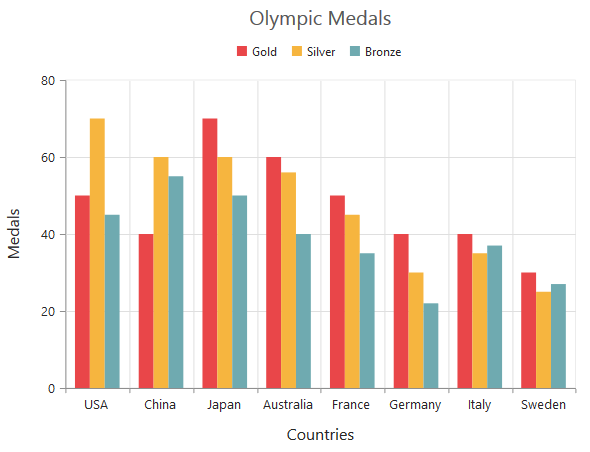### Place labels on ticks

Labels in the category axis can be placed on ticks by setting the LabelPlacement property of axis to onTicks. Since the default value of the LabelPlacement property is betweenTicks, labels are placed between the ticks.

• CSHTML
• ``````<ej-chart id="chartContainer">
// ...

<e-primary-x-axis label-placement="onTicks"></e-primary-x-axis>

// ...

</ej-chart>``````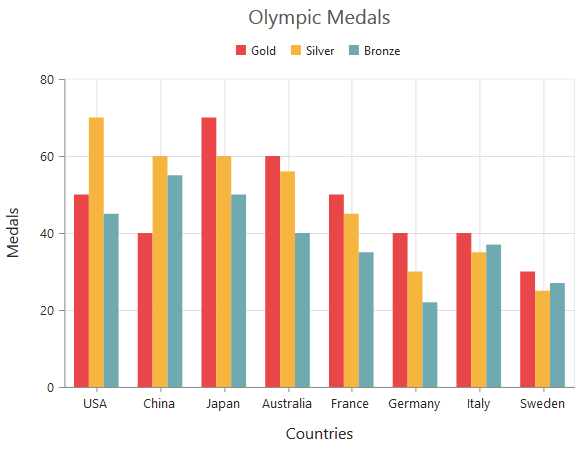### Display labels after a fixed interval

To display the labels after a fixed interval n, set the Interval property of the axis range as n. The default value of the interval is 1. So, all the labels are displayed.

• CSHTML
• ``````<ej-chart id="chartContainer">
// ...

<e-primary-x-axis><e-range interval="2"></e-range></e-primary-x-axis>

// ...

</ej-chart>``````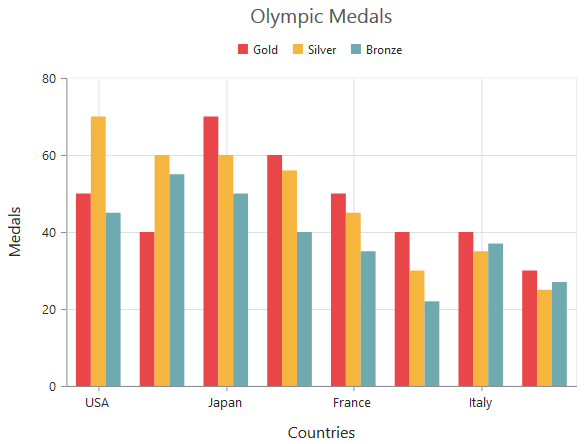### Indexed Category Axis

Category axis can also plot points based on index value of data points. Index based plotting can be enabled by setting IsIndexed property to true in the axis.

• CSHTML
• ``````<ej-chart id="chartContainer">
<e-primary-x-axis is-indexed="true"></e-primary-x-axis>
<e-chart-series>
<e-series>
<e-points>
<e-point x="Monday" y="50"></e-point>
<e-point x="Tuesday" y="40"></e-point>
<e-point x="Wednesday" y="70"></e-point>
<e-point x="Thursday" y="60"></e-point>
<e-point x="Friday" y="50"></e-point>
<e-point x="Monday" y="40"></e-point>
<e-point x="Monday" y="30"></e-point>
</e-points>
</e-series>
</e-chart-series>
</ej-chart>``````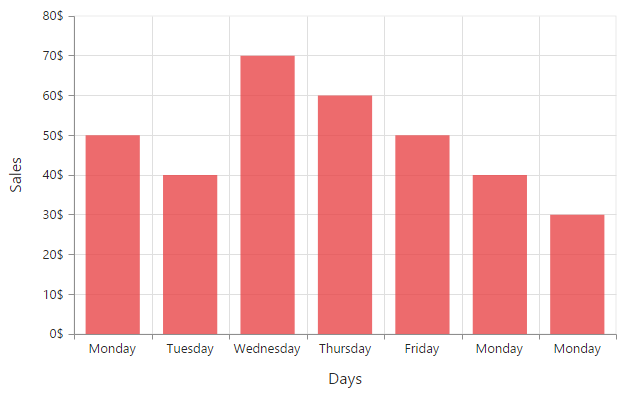While Category axis IsIndexed value false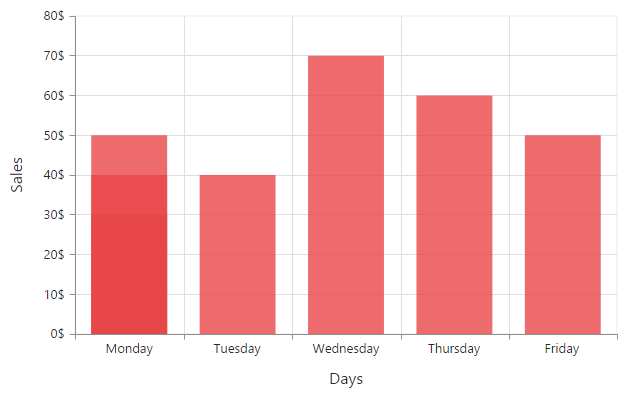## Numeric Axis

Numeric axis supports numerical scale, and displays numbers as labels. To use numeric axis, set the ValueType property of the axis to Double.

• CSHTML
• ``````<ej-chart id="container">
// ...
<e-primary-x-axis value-type="@AxisValueType.Double"></e-primary-x-axis>
// ...
</ej-chart>``````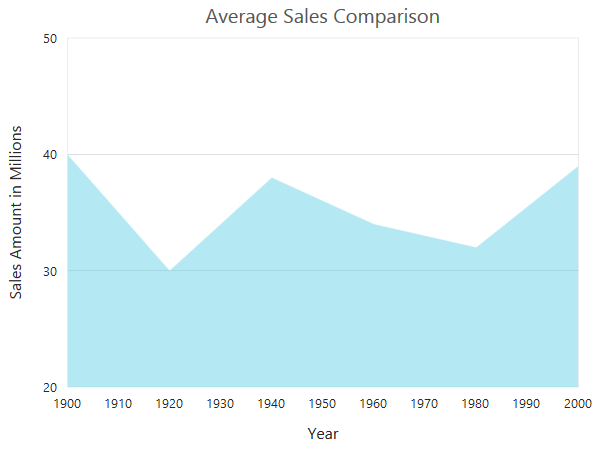### Customize numeric range

To customize the range of an axis, use the Range property. By using this, you can set Minimum, Maximum, and Interval values. Nice range is calculated automatically based on the provided data, by default.

• CSHTML
• ``````<ej-chart id="chartContainer">
<e-primary-y-axis><e-range min="0" max="50"></e-range></e-primary-y-axis>
</ej-chart>``````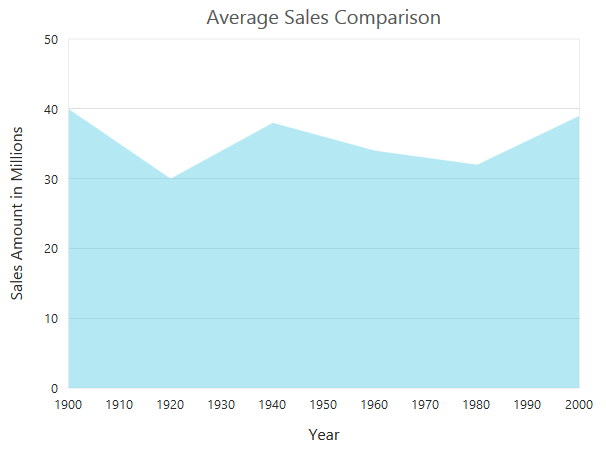#### Customizing numeric interval

Axis interval can be customized by using the Interval property of the axis range. Nice interval is calculated based on the minimum and maximum values of the provided data, by default.

• CSHTML
• ``````<ej-chart id="chartContainer">
<e-primary-y-axis><e-range interval="5"></e-range></e-primary-y-axis>
</ej-chart>``````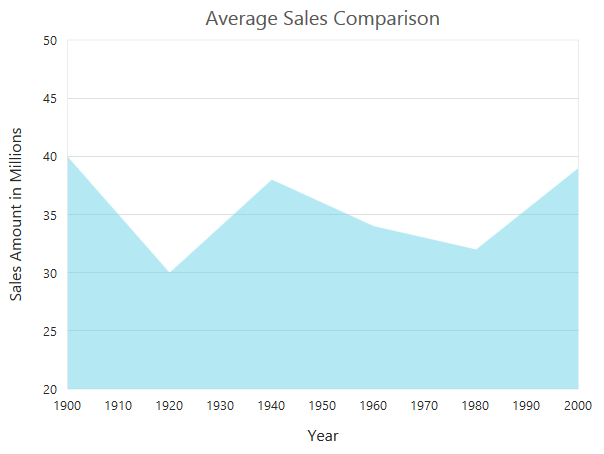### Apply padding to the range

Padding can be applied to the minimum and maximum values of the axis range by using the RangePadding property. Numeric axis supports the following types of padding:

• None
• Round
• Normal

None

When the value of RangePadding property is None, padding can not be applied to the axis. This is also the default value of the RangePadding.

• CSHTML
• ``````<ej-chart id="chartContainer">
</ej-chart>``````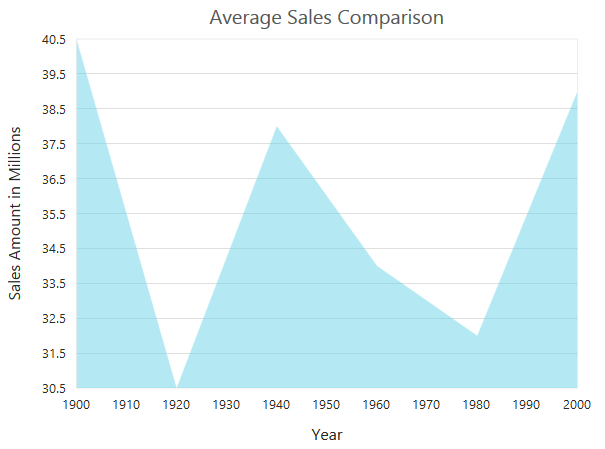#### Round

When the value of RangePadding property is Round, the axis range will be rounded to the nearest possible value and it will be divided by the interval.

• CSHTML
• ``````<ej-chart id="chartContainer">
</ej-chart>``````

Chart before rounding axis rangeChart after rounding axis range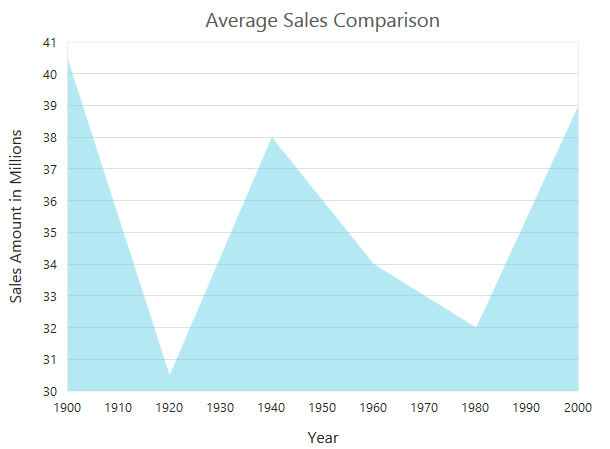When the value of RangePadding property is Additional, the axis range will be rounded and an interval of the axis will be added as padding to the minimum and maximum values of the range.

• CSHTML
• ``````<ej-chart id="chartContainer">
</ej-chart>``````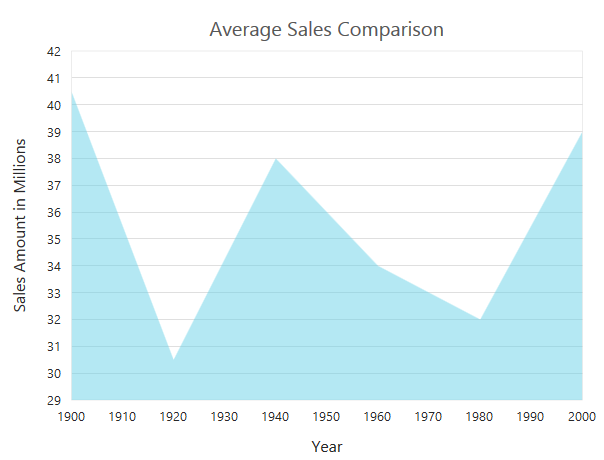Normal

When the value of the RangePadding property is Normal, the padding will be applied to the axis based on the range calculation.

• CSHTML
• ``````<ej-chart id="chartContainer">
</ej-chart>``````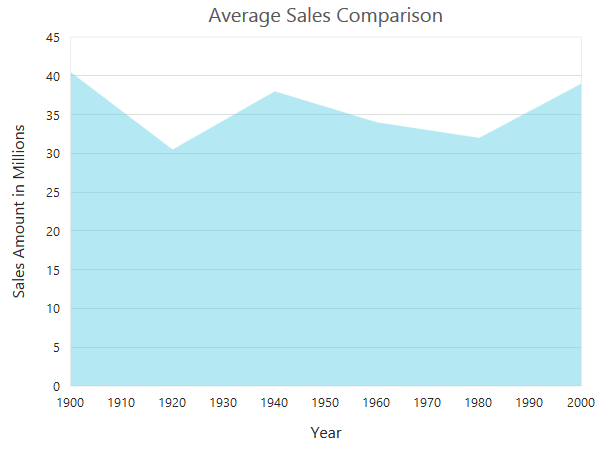### Customizing the starting range of the axis

By default, the Y axis value will be calculated from 0 for column, bar, stacking column, and stacking bar series types. You can modify this behavior by setting the StartFromZero property to false in the axis. When setting this, the axis minimum value will be calculated based on the value for data points.

• CSHTML
• ``````<ej-chart id="chartContainer">
</ej-chart>``````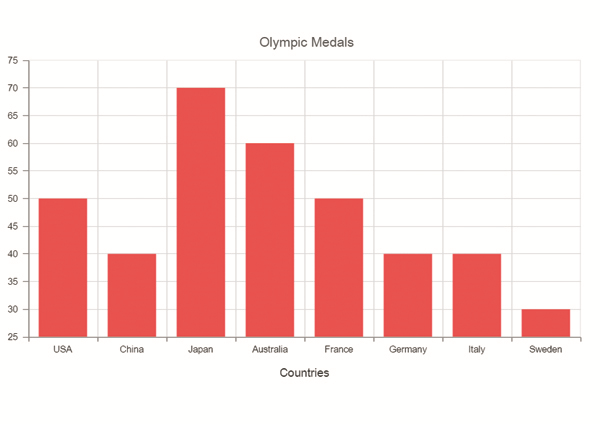## DateTime Axis

Date time axis supports date time scale, and displays the date time values as axis labels in the specified format. To use date time axis, set the ValueType property of the axis to Datetime.

• CSHTML
• ``````<ej-chart id="container">
// ...
<e-primary-x-axis value-type="@AxisValueType.Datetime"></e-primary-x-axis>
// ...
</ej-chart>``````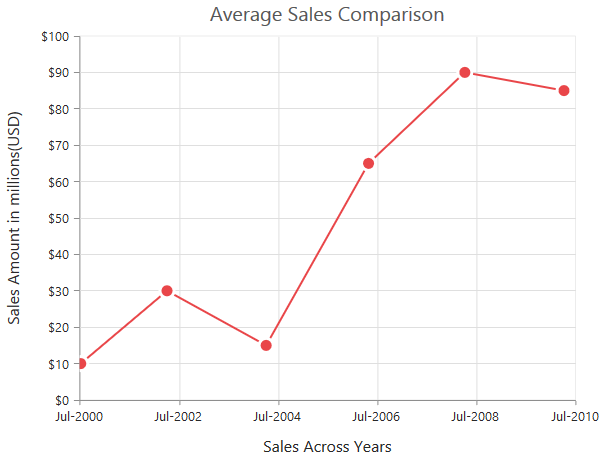### Customizing date time range

Axis range can be customized by using the Range property. By using this, you can customize Minimum, Maximum, and Interval values. Nice range is calculated automatically based on the provided data, by default.

• CSHTML
• ``````<ej-chart id="chartContainer">
<e-primary-x-axis><e-range min="2000/6/1" max="2010/6/1"></e-range></e-primary-x-axis>
</ej-chart>``````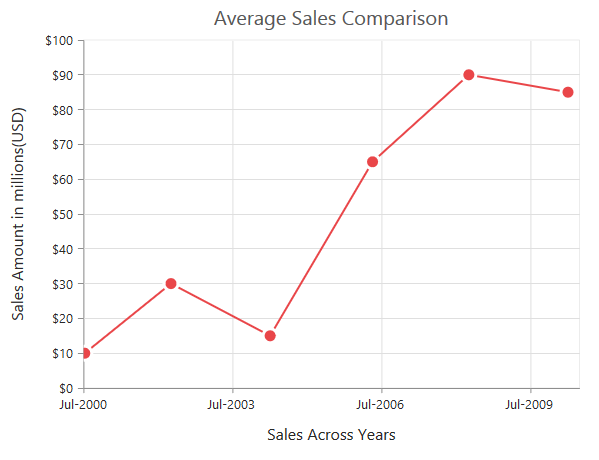### Date time intervals

Date time intervals can be customized by using the Interval and IntervalType properties of the axis. For example, when you set Interval as 2 and IntervalType as Years, it considers 2 years as interval.

Essential chart supports the following types of interval for date time axis:

• Days
• Hours
• Milliseconds
• Minutes
• Months
• Seconds
• Years
• CSHTML
• ``````<ej-chart id="container">
// ...
<e-primary-x-axis interval-type="@ChartIntervalType.Years"><e-range interval="2"></e-range></e-primary-x-axis>
// ...
</ej-chart>``````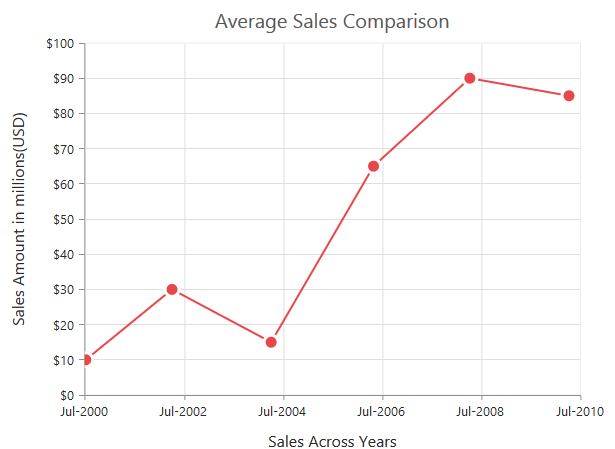### Apply padding to the range

Padding can be applied to the minimum and maximum values of the range by using the RangePadding property. Date time axis supports the following types of padding:

• None
• Round

None

When the value of the RangePadding property is None, padding will be applied to the axis. This is also the default value of the rangePadding.

• CSHTML
• ``````<ej-chart id="chartContainer">
</ej-chart>``````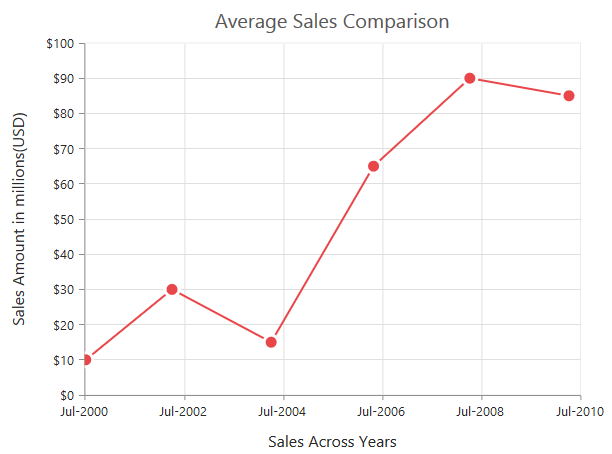Round

When the value of RangePadding property is Round, the axis range will be rounded to the nearest possible date time value.

• CSHTML
• ``````<ej-chart id="chartContainer">
</ej-chart>``````

Chart before rounding axis range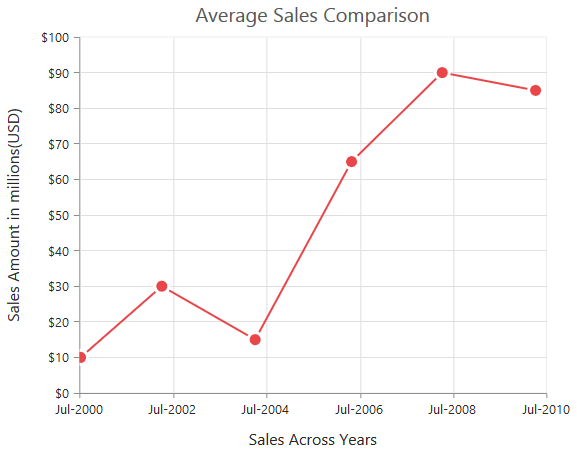Chart after rounding axis range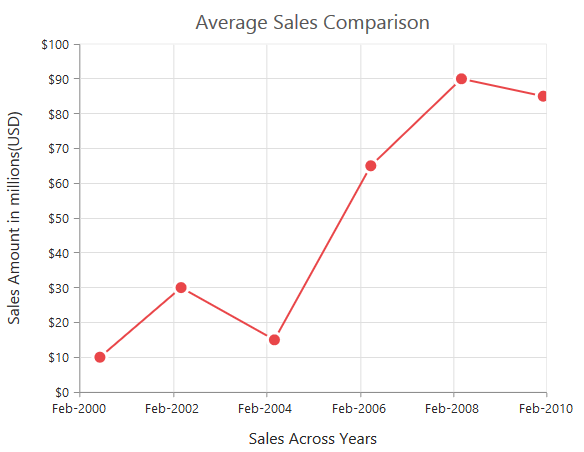When the value of the RangePadding property is Additional, the range will be rounded, and date time intervals of the axis are added as padding to the minimum and maximum values of the range.

• CSHTML
• ``````<ej-chart id="chartContainer">
</ej-chart>``````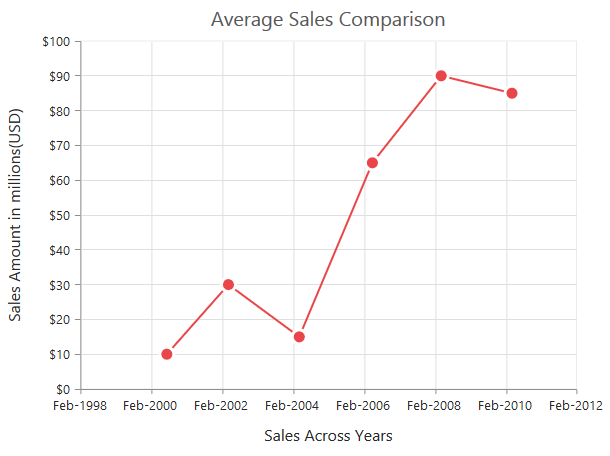## DateTime Category Axis

DateTime category axis takes date time value as input, but behaves like category axis. This is used to display the date time values with nonlinear intervals (used to depict the business days by skipping holidays). To use date time axis, set the ValueType property of the axis to DatetimeCategory.

• CSHTML
• ``````<ej-chart id="container">
// ...
<e-primary-x-axis value-type="@AxisValueType.DateTimeCategory"></e-primary-x-axis>
// ...
</ej-chart>``````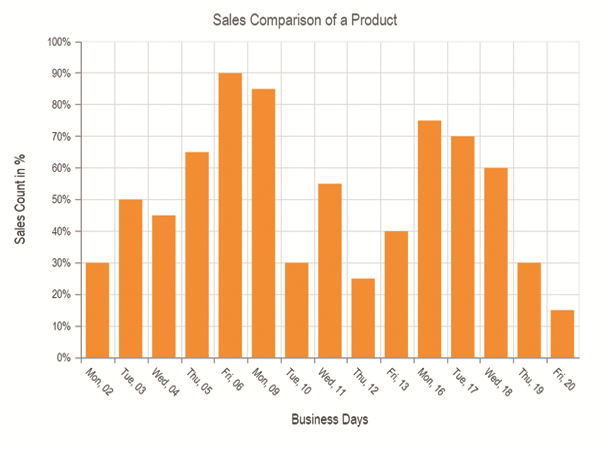### Customizing DateTime Category range

Axis range can be customized by using the range property. By using this, you can customize Minimum, Maximum, and Interval values. Datetime category axis takes numeric input for minimum and maximum properties.

• CSHTML
• ``````<ej-chart id="container">
// ...
<e-primary-x-axis><e-range min="0" max="4"></e-range></e-primary-x-axis>
// ...
</ej-chart>``````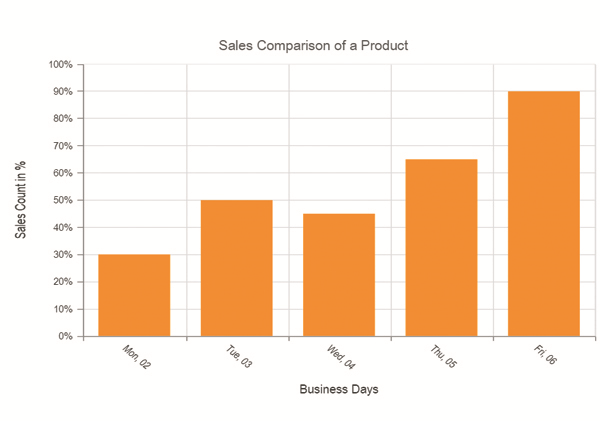### DateTime category intervals

Date time category intervals can be customized by using the Interval and IntervalType properties of the axis. For example, when you set the intervalType as months, it displays only the first label of all the months from the data.

Essential Chart supports the following types of interval for date time category axis:

• Days
• Hours
• Milliseconds
• Minutes
• Months
• Seconds
• Years
• Auto
• CSHTML
• ``````<ej-chart id="container">
// ...
<e-primary-x-axis interval-type="@ChartIntervalType.Months"></e-primary-x-axis>
// ...
</ej-chart>``````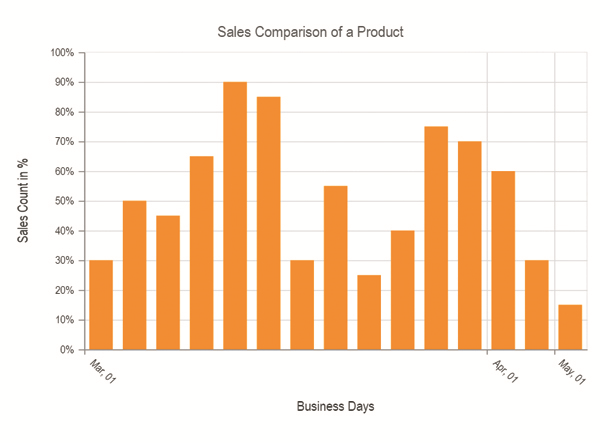## Logarithmic Axis

Logarithmic axis supports logarithmic scale, and it is used in visualizing when the data has values with both lower (eg: 10-6) and higher (eg: 106) order of magnitudes. To use logarithmic axis, set the ValueType property of the axis to Logarithmic.

• CSHTML
• ``````<ej-chart id="container">
// ...
<e-primary-x-axis value-type="@AxisValueType.Logarithmic"></e-primary-x-axis>
// ...
</ej-chart>``````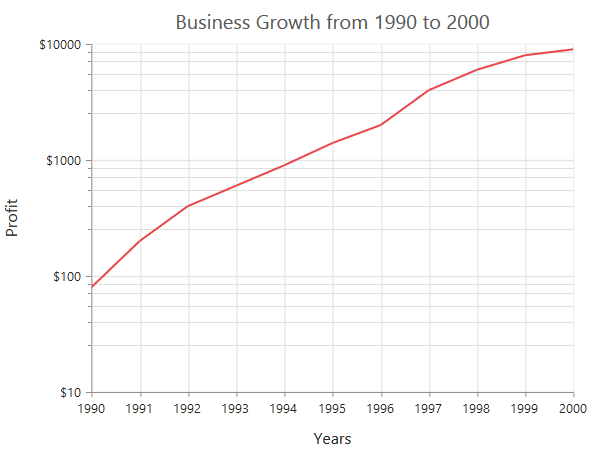### Customize logarithmic range

Logarithmic range can be customized by using the Range property. By using this, you can customize Minimum, Maximum, and Interval values. Nice range is calculated automatically based on the provided data, by default.

• CSHTML
• ``````<ej-chart id="container">
// ...
<e-primary-x-axis><e-range min="1" max="5"></e-range></e-primary-x-axis>
// ...
</ej-chart>``````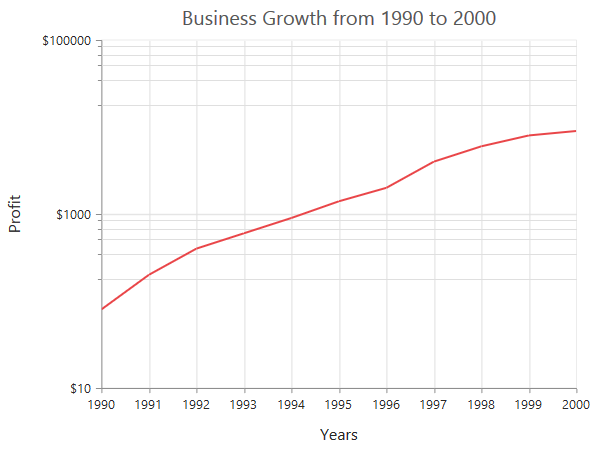### Logarithmic base

Logarithmic base can be customized by using the LogBase property of the axis. The default value of the LogBase is 10.

• CSHTML
• ``````<ej-chart id="container">
// ...
<e-primary-x-axis log-base="2"></e-primary-x-axis>
// ...
</ej-chart>``````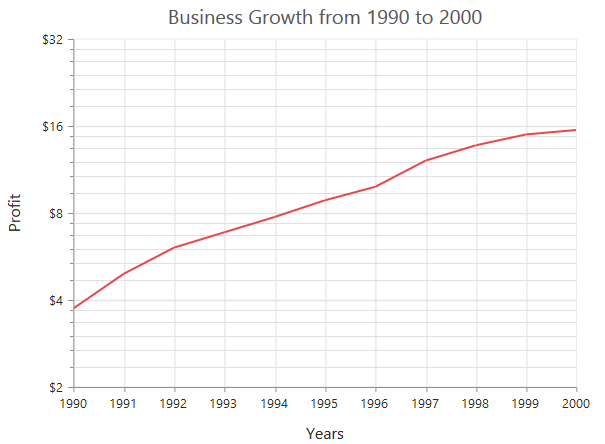### Logarithmic interval

Logarithmic axis interval can be customized by using the Interval property of the axis. When the logarithmic base is 10 and logarithmic interval is 2, the axis labels are placed at an interval of 102. The default value of the interval is 1.

• CSHTML
• ``````<ej-chart id="container">
// ...
<e-primary-x-axis><e-range interval="2"></e-range></e-primary-x-axis>
// ...
</ej-chart>``````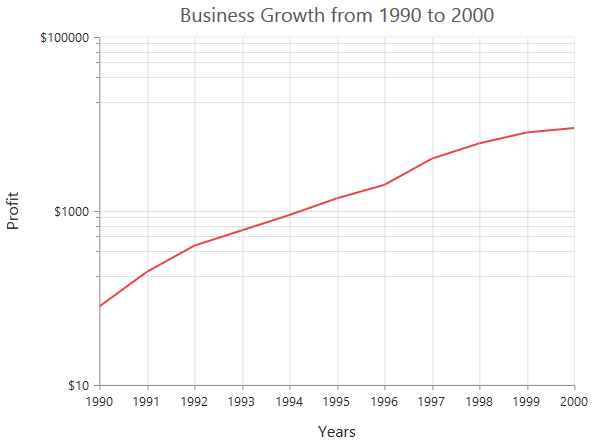## Label Format

### Format numeric labels

Numeric labels can be formatted by using the LabelFormat property. Numeric values can be formatted with n (number with decimal points), c (currency), and p (percentage) commands.

• CSHTML
• ``````<ej-chart id="container">
// ...
<e-primary-x-axis label-format="c"></e-primary-x-axis>
// ...
</ej-chart>``````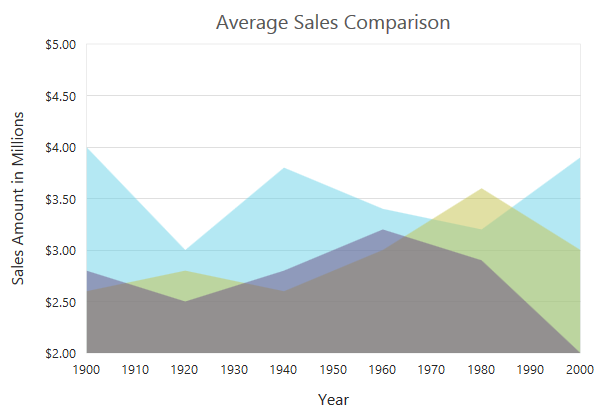The following table describes the result of some commonly used label formats on numeric values.

 Label value Label format property value Result Description 1000 n1 1000.0 The number is rounded to 1 decimal place 1000 n2 1000.00 The number is rounded to 2 decimal places 1000 n3 1000.000 The number is rounded to 3 decimal places 0.01 p1 1.0% The number is converted to percentage with 1 decimal place 0.01 p2 1.00% The number is converted to percentage with 2 decimal places 0.01 p3 1.000% The number is converted to percentage with 3 decimal places 1000 c1 \$1,000.0 The dollar symbol is appended to number, and the number is rounded to 1 decimal place 1000 c2 \$1,000.00 The dollar symbol is appended to number, and the number is rounded to 2 decimal places

### Format date time values

Date time labels can be formatted by using the LabelFormat property of the axis.

• CSHTML
• ``````<ej-chart id="container">
// ...
<e-primary-x-axis label-format="dd/MMMM/yyyy"></e-primary-x-axis>
// ...
</ej-chart>``````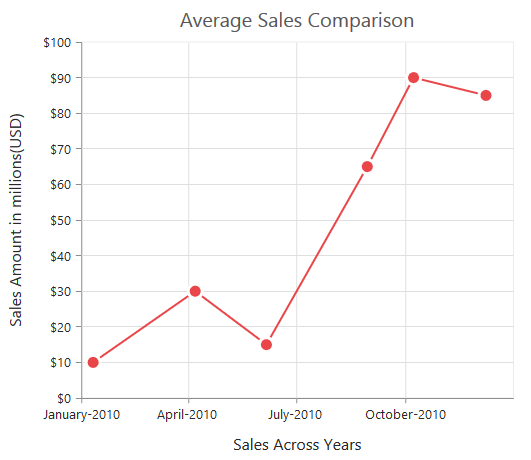The following table describes the result some common date time formats to the labelFormat property.

 Label value Label format property Value Result Description new Date(2000, 03, 10) dddd Monday The date is displayed in day format new Date(2000, 03, 10) MM/dd/yyyy 04/10/2000 The date is displayed in month/date/year format new Date(2000, 03, 10) n3 1000.000 The number is rounded to 3 decimal places new Date(2000, 03, 10) MMM Apr The shorthand month for the date is displayed new Date(2000, 03, 10) t 12:00 AM Time of the date value is displayed as label new Date(2000, 03, 10) hh:mm:ss 12:00:00 The label is displayed in hours:minutes:seconds format

### Custom label format

Prefix and suffix can be added to the category labels by using the LabelFormat property. You can use the {value} as placeholder text in your custom text, it is replaced with the corresponding axis label at the runtime.

• CSHTML
• ``````<ej-chart id="container">
// ...
<e-primary-x-axis label-format="\${value} K"></e-primary-x-axis>
// ...
</ej-chart>``````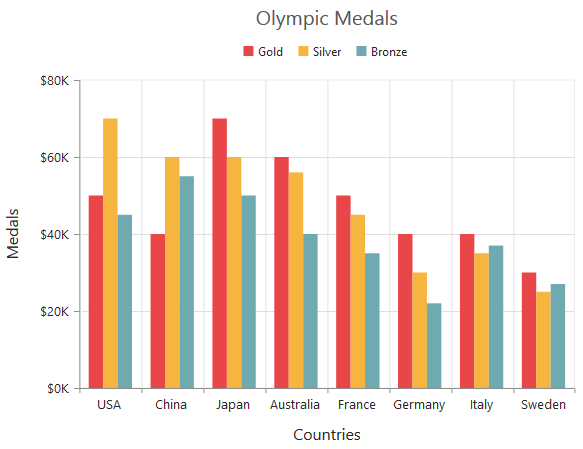## Common axis features

Customization of features such as axis title, labels, grid lines, and tick lines are common to all the axis. Each of these features have been explained in this section.

### Axis crossing

Axis can be positioned anywhere in the chart area by using the CrossesAt property of axis. This property specifies where the horizontal axis should intersect or cross the vertical axis and vice-versa. Default value of CrossesAt property is null.

• CSHTML
• ``````<ej-chart id="container">
// ...
<e-primary-x-axis crosses-at="0"></e-primary-x-axis>
// ...
</ej-chart>``````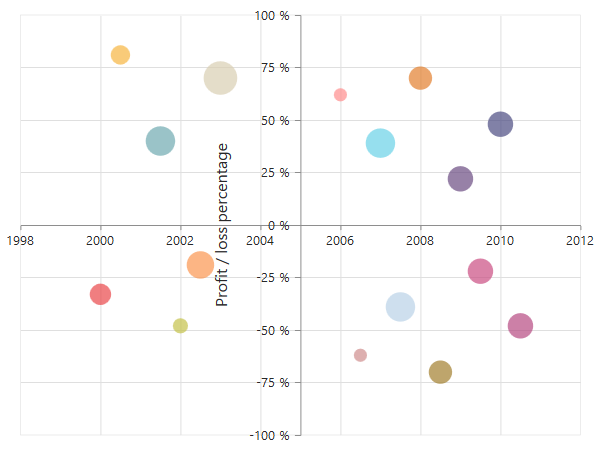#### Crossing a specific Axis

The CrossesInAxis property takes axis name as input and determines the axis used for crossing. By default, all the horizontal axes cross in primary Y axis, and all the vertical axes cross in primary X axis.

• CSHTML
• ``````<ej-chart id="container">
// ...
<e-primary-x-axis crosses-at="-0.2" crosses-in-axis="SecondaryYAxis"></e-primary-x-axis>
// ...
</ej-chart>``````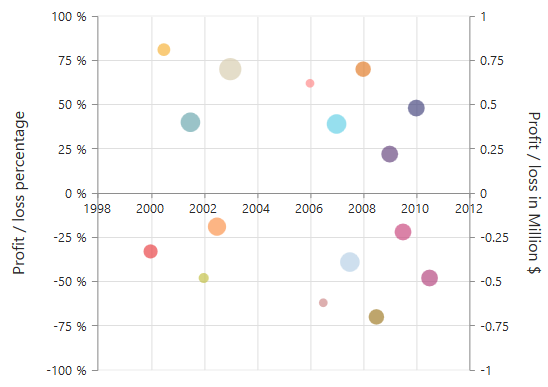Axis will be placed in the opposite side if value of CrossesAt property is greater than the maximum value of crossing axis (axis name provided through CrossesInAxis property or primary Y axis for horizontal axis).

• CSHTML
• ``````<ej-chart id="container">
// ...
<e-primary-x-axis crosses-at="200"></e-primary-x-axis>
// ...
</ej-chart>``````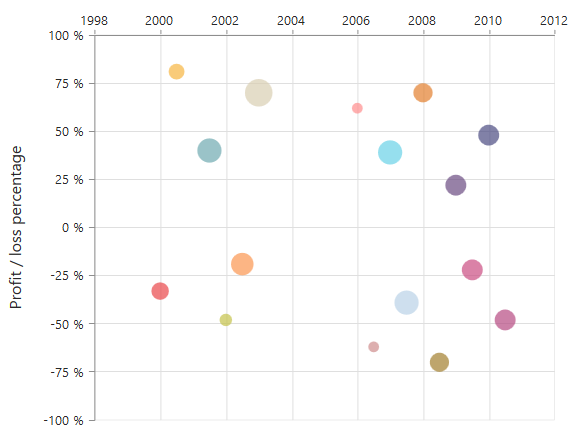#### Positioning the axis elements while crossing

The `ShowNextToAxisLine` property is used for controlling the axis elements movement along with the axis line while axis crossing is performed. When the showNextToAxisLine is set to false, the axis lines and the tick lines are placed at the crossing value, and the axis elements will be placed outside the chart area. The default value of `ShowNextToAxisLine` is true.

• CSHTML
• ``````<ej-chart id="container">
// ...
<e-primary-x-axis crosses-at="0" show-next-to-axis-line="false"></e-primary-x-axis>
// ...
</ej-chart>``````

The axis is placed at the crossing value without the axis elements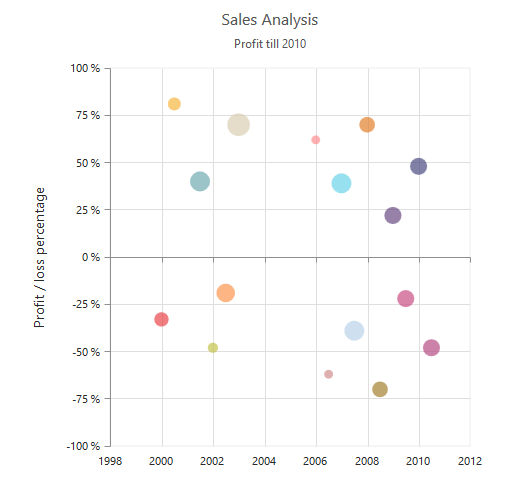### Axis Visibility

Axis visibility can be controlled by using the Visible property of the axis. The default value of the Visible property is true.

• CSHTML
• ``````<ej-chart id="container">
// ...
<e-primary-y-axis visible="false"></e-primary-y-axis>
// ...
</ej-chart>``````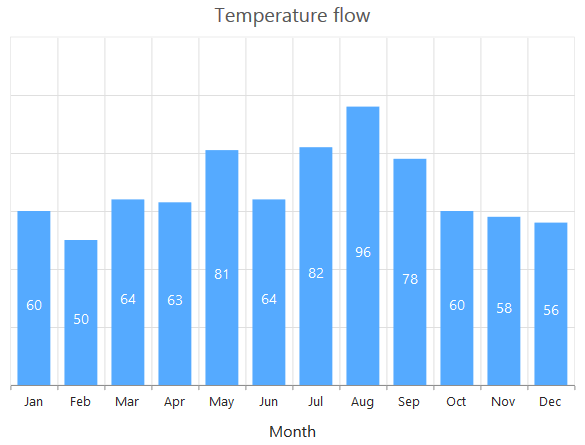### Axis title

The Title property in the axis provides options to customize the text and font of the axis title. Axis does not display the title, by default. Title text can also be trimmed based on the title text’s length or specified length.

• CSHTML
• ``````<ej-chart id="container">
// ...
<e-primary-x-axis>
<e-title enable-trim="true" maximum-title-width="80" text="Month">
<e-font font-weight="Bold" font-family="Segoe UI" font-size="16px"></e-font>
</e-title>
</e-primary-x-axis>
// ...
</ej-chart>``````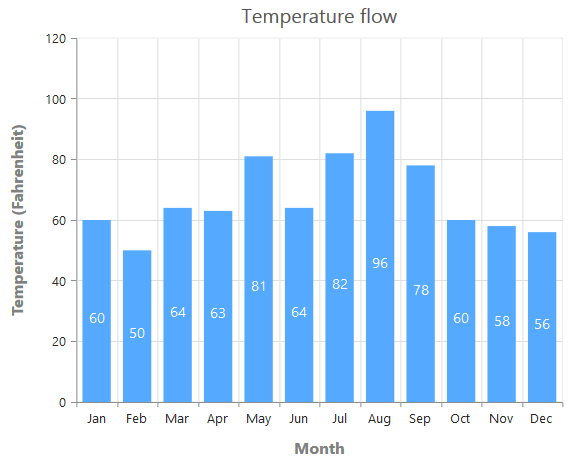You can modify the position of the axis title either inside or outside the chart area by using the Position property. By default, it will be placed outside the chart area. In addition, you can also change the alignment of the title to near, far, or center by using Alignment property. By using Offset property, you can change the position with respect to pixels.

• CSHTML
• ``````<ej-chart id="container">
// ...
<e-primary-x-axis axis-title-position="Inside" alignment="Near" plot-offset="10">
</e-primary-x-axis>
// ...
</ej-chart>``````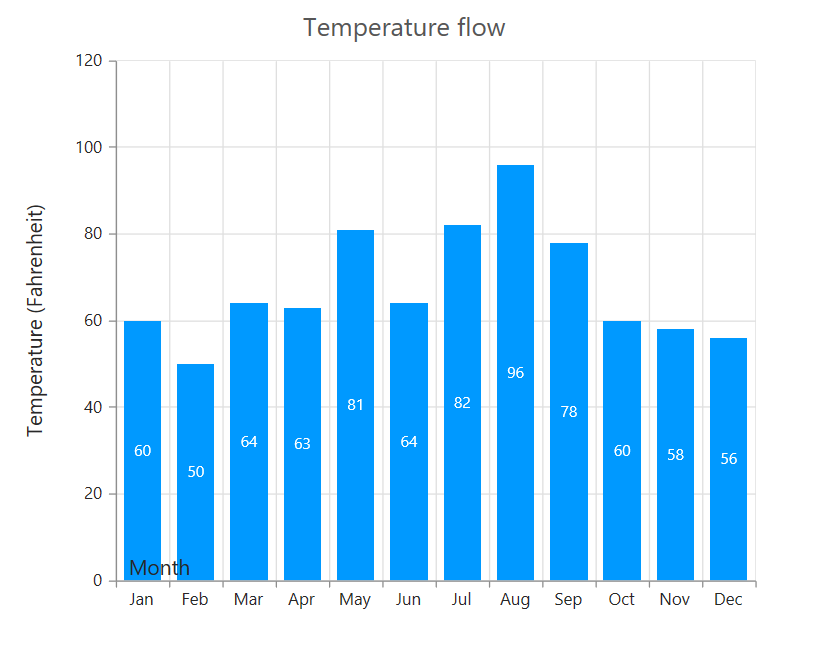### Label customization

The Font property of the axis provides options to customize the FontFamily, color, opacity, size, and FontWeight of the axis labels.

• CSHTML
• ``````<ej-chart id="container">
// ...
<e-primary-x-axis>
<e-font color="Blue" font-weight="Bold" font-family="Segoe UI" font-size="14px"></e-font>
</e-primary-x-axis>
// ...
</ej-chart>``````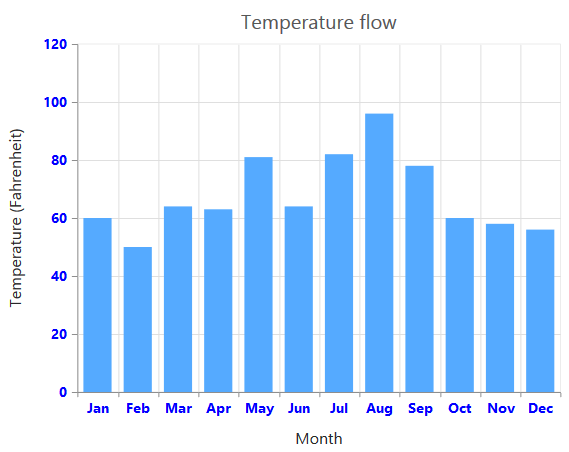### Label and tick positioning

Axis labels and ticks can be positioned inside or outside the chart area by using the LabelPosition and TickPosition properties. The labels and ticks are positioned outside the chart area, by default.

• CSHTML
• ``````<ej-chart id="container">
// ...
<e-primary-x-axis axislabel-position="Inside" tick-lines-position="Inside">
</e-primary-x-axis>
// ...
</ej-chart>``````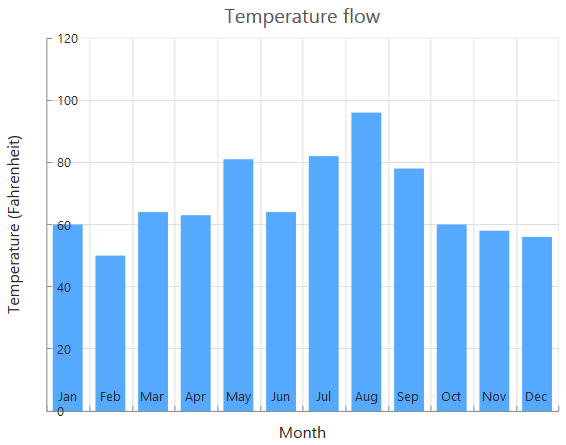### Edge labels placement

Labels with long text at the edges of an axis may appear partially outside the chart. By using the EdgeLabelPlacement property, you can avoid the partial appearance of the labels at the corners.

• CSHTML
• ``````<ej-chart id="container">
// ...
<e-primary-x-axis edge-label-placement="Shift">
</e-primary-x-axis>
// ...
</ej-chart>``````

Chart before setting edge label placement to X-axis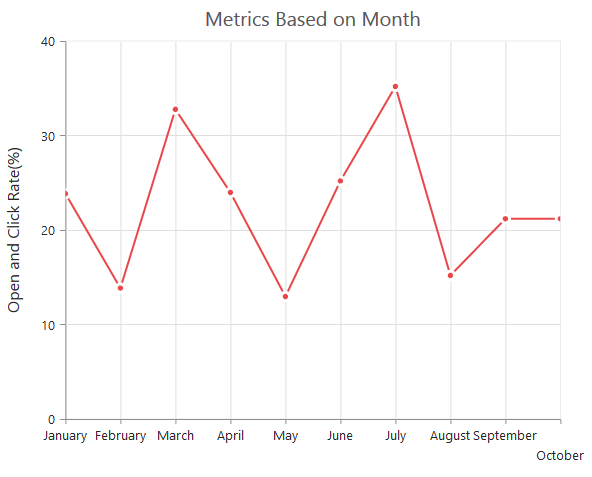Chart after setting edge label placement to X-axis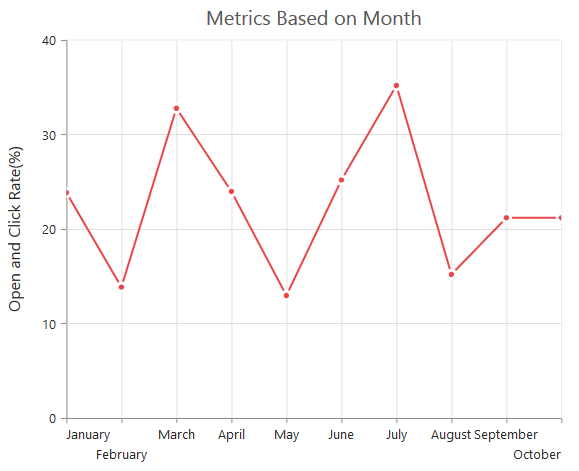### Grid lines customization

The MajorGridLines and MinorGridLines properties in the axis are used to customize the major grid lines and minor grid lines of an axis. They provide options to change the width, color, visibility, and opacity of the grid lines. The minor grid lines are not visible, by default.

• CSHTML
• ``````<ej-chart id="container">
// ...
<e-primary-x-axis minor-ticks-per-interval="0">
<e-major-grid-lines color="Blue" visible="true" width="1"></e-major-grid-lines>
<e-minor-grid-lines color="red" visible="false" width="1"></e-minor-grid-lines>
</e-primary-x-axis>
// ...
</ej-chart>``````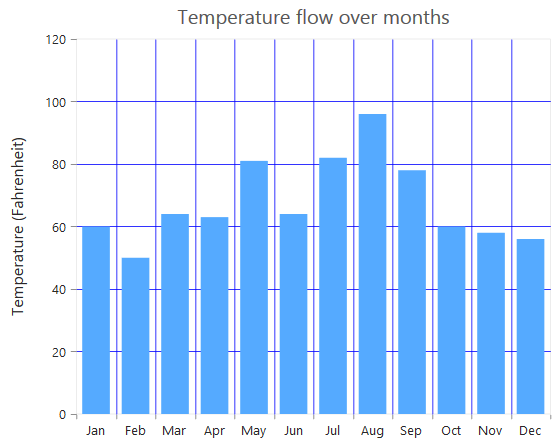### Tick lines customization

The MajorTickLines and MinorTickLines properties in the axis are used to customize the major and minor tick lines of an axis. They provide options to change the width, size, color, and visibility of the grid lines. The minor tick lines are not visible, by default.

• CSHTML
• ``````<ej-chart id="container">
// ...
<e-primary-x-axis minor-ticks-per-interval="0">
<e-major-tick-lines color="Blue" visible="true" width="1" size="5"></e-major-tick-lines>
<e-minor-tick-lines color="red" visible="false" width="1" size="5"></e-minor-tick-lines>
</e-primary-x-axis>
// ...
</ej-chart>``````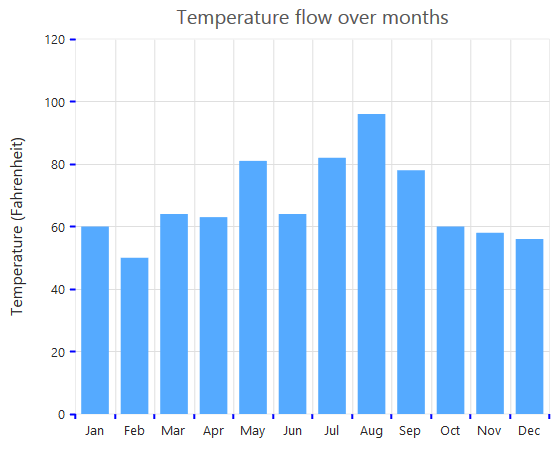### Inversing axis

Axis can be inversed by using the IsInversed property of the axis. The default value of the IsInversed property is false.

• CSHTML
• ``````<ej-chart id="container">
// ...
<e-primary-x-axis is-inversed="false">
</e-primary-x-axis>
// ...
</ej-chart>``````

Chart before inversing the axes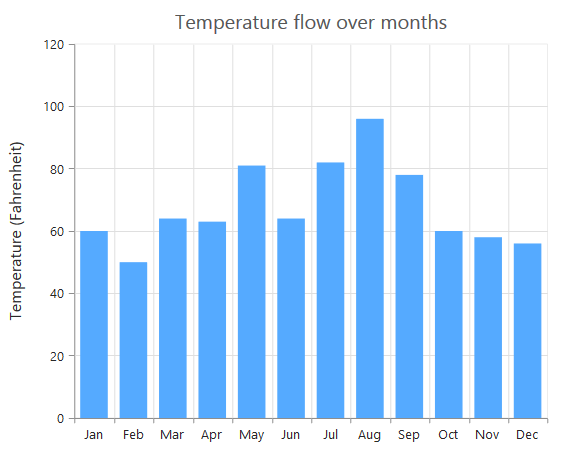Chart after inversing the axes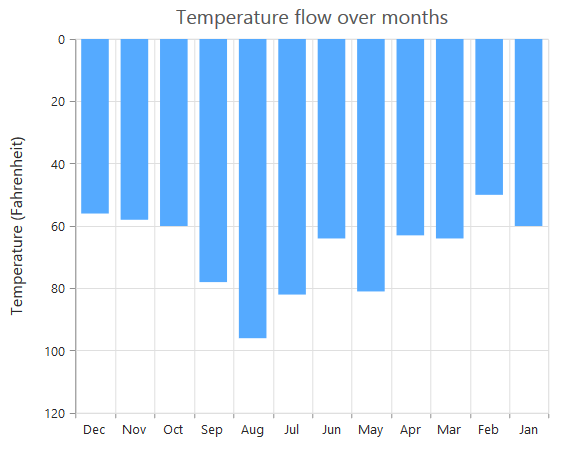### Place axes at the opposite side

The OpposedPosition property of axis can be used to place the axis at the opposite side of its default position. The default value of the OpposedPosition property is false.

• CSHTML
• ``````<ej-chart id="container">
// ...
<e-primary-x-axis opposed-position="true">
</e-primary-x-axis>
// ...
</ej-chart>``````

Chart with X and Y axes at normal position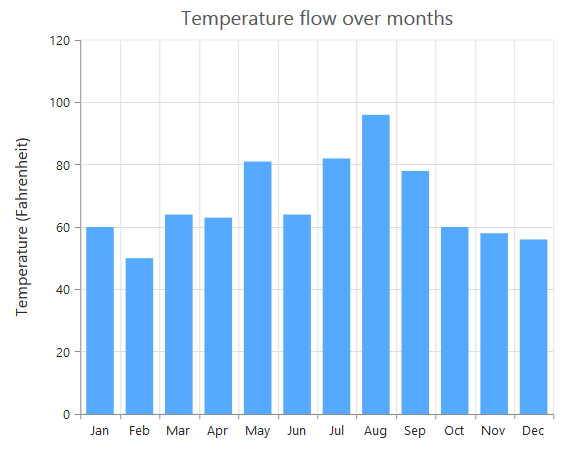Chart with Y-axis at opposed position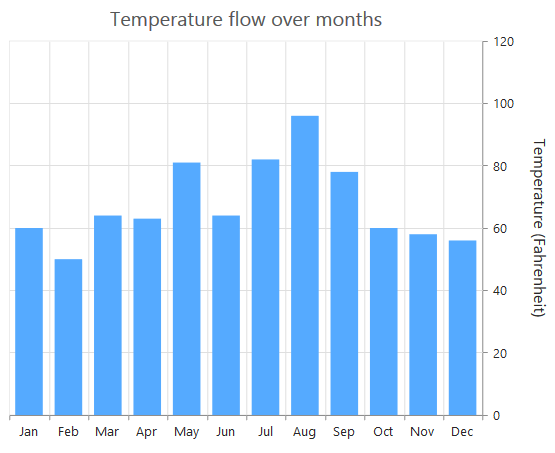### Maximum number of labels per 100 pixels

Maximum of 3 labels are displayed for each 100 pixels in the axis, by default. The maximum number of labels, which present within the 100 pixels length can be customized by using the MaximumLabels property of the axis. This property is applicable only for an automatic range calculation, and it does not work when you set the value for Interval property in axis range.

• CSHTML
• ``````<ej-chart id="container">
// ...
<e-primary-x-axis maximum-labels="1">
</e-primary-x-axis>
// ...
</ej-chart>``````

Chart before setting maximum labels per 100 pixels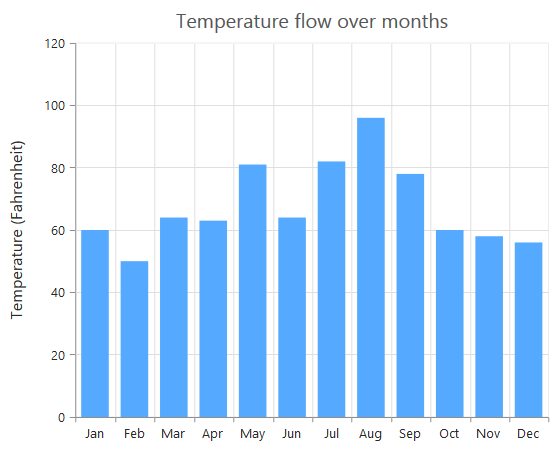Chart after setting maximum labels one per 100 pixels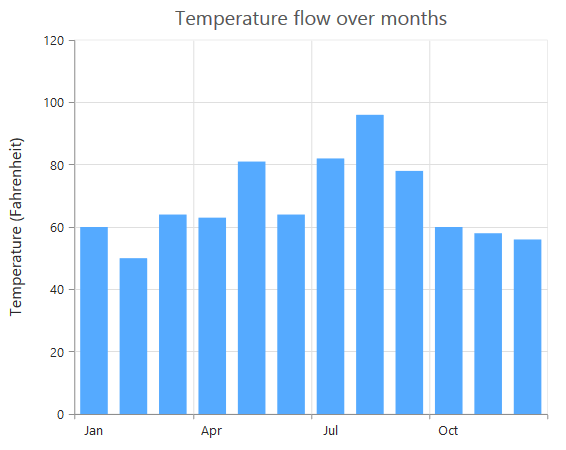## Multiple Axis

Multiple axes can be used in the chart, and chart area can be split into multiple panes to draw multiple series with multiple axes.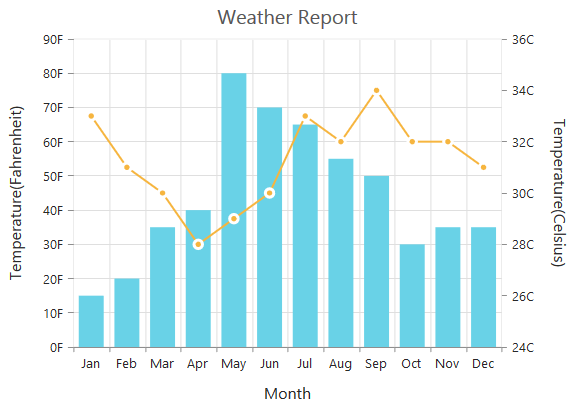An additional horizontal or vertical axis can be added to the chart by adding an axis instance to the Axes collection. You can associate it to a series by specifying name of the axis to the XAxisName or YAxisName property of the series.

• CSHTML
• ``````<ej-chart id="container">
// ...
<e-axes>
<e-axis name="SecondaryX"></e-axis>
<e-axis name="SecondaryY"></e-axis>
</e-axes>
<e-chart-series>
<e-series x-axis-name="SecondaryX" y-axis-name="SecondaryY"></e-series>
</e-chart-series>
// ...
</ej-chart>``````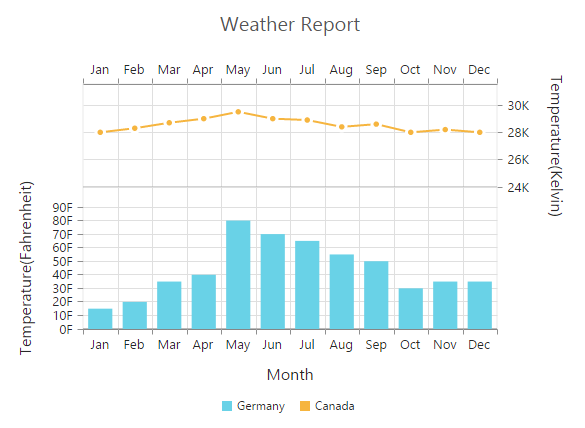## Smart axis labels

When the Axis labels overlap with each other based on the chart dimensions and label size, use the LabelIntersectAction property of the axis to avoid overlapping. The default value of the LabelIntersectAction is None. The other available values of the label intersect actions are Rotate45, Rotate90, Trim, MultipleRows, Wrap, and Hide.

• CSHTML
• ``````<ej-chart id="container">
// ...
<e-primary-x-axis label-intersect-action="MultipleRows"></e-primary-x-axis>
// ...
</ej-chart>``````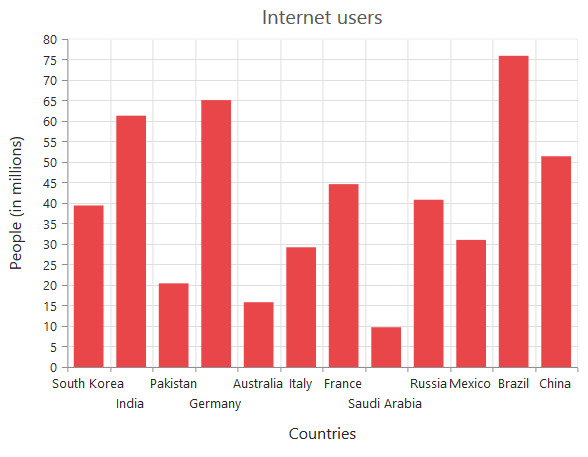The following screenshot displays the result when the LabelIntersectAction property is set to Rotate45.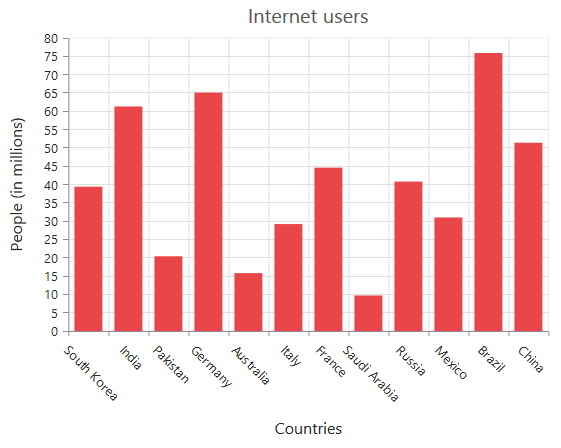The following screenshot displays the result when the LabelIntersectAction property is set to Rotate90.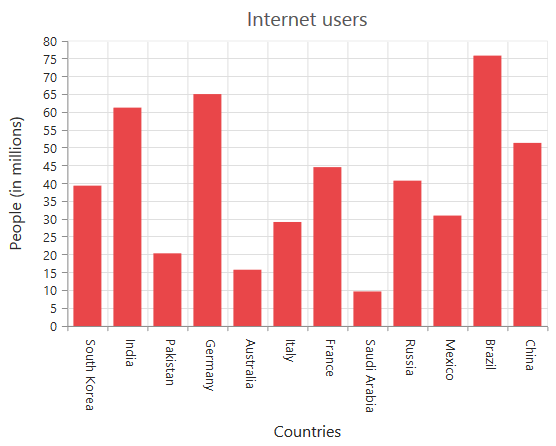The following screenshot displays the result when the LabelIntersectAction property is set to Wrap.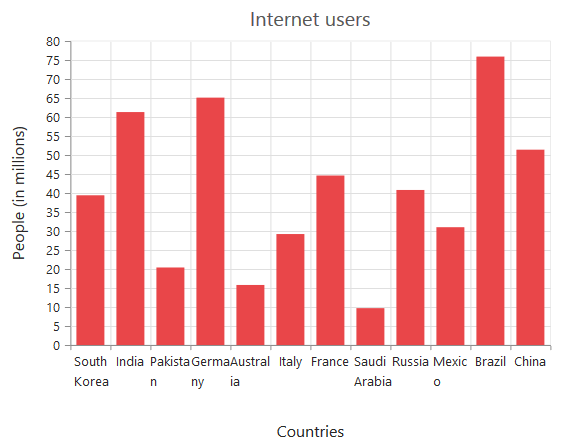The following screenshot displays the result when the LabelIntersectAction property is set to trim.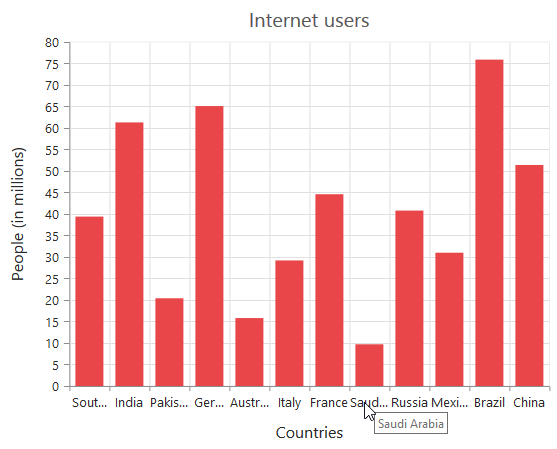The following screenshot displays the result when the LabelIntersectAction property is set to Hide.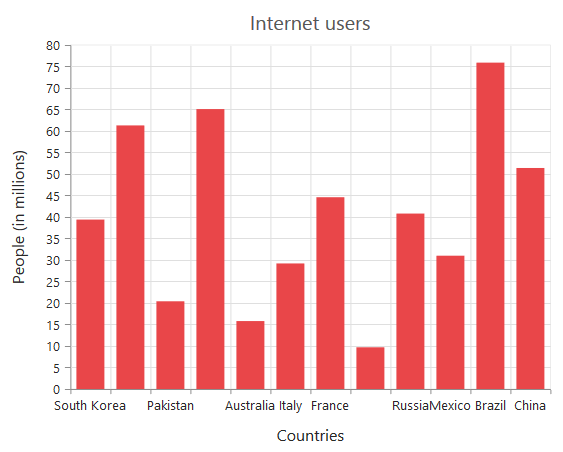The following screenshot displays the result when the LabelIntersectAction property is set to MultipleRows.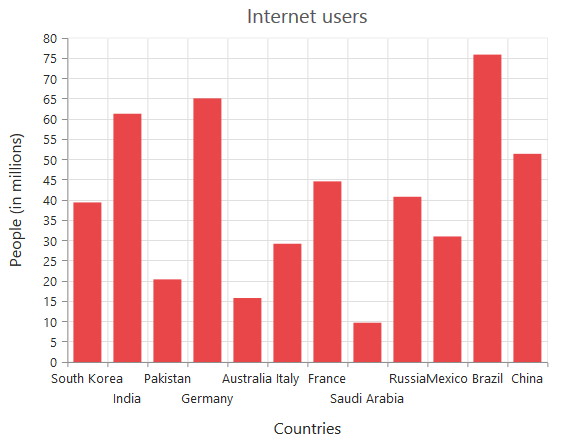The following screenshot displays the result when the LabelIntersectAction property is set to WrapByWord.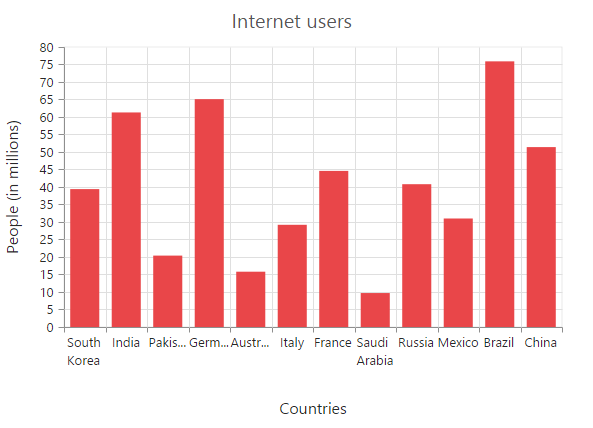## Multi-level Labels

Axis can be customized with multiple levels of labels by using the MultiLevelLabels property. These labels are placed based on the start and end range values. You can add any number of labels to an axis.

• CSHTML
• ``````<ej-chart id="container">
// ...
<e-primary-x-axis>
<e-multi-level-labels>
<e-multi-level-label visible="true" text="Quater 1" start="-0.5" end="2.5"></e-multi-level-label>
</e-multi-level-labels>
</e-primary-x-axis>
// ...
</ej-chart>``````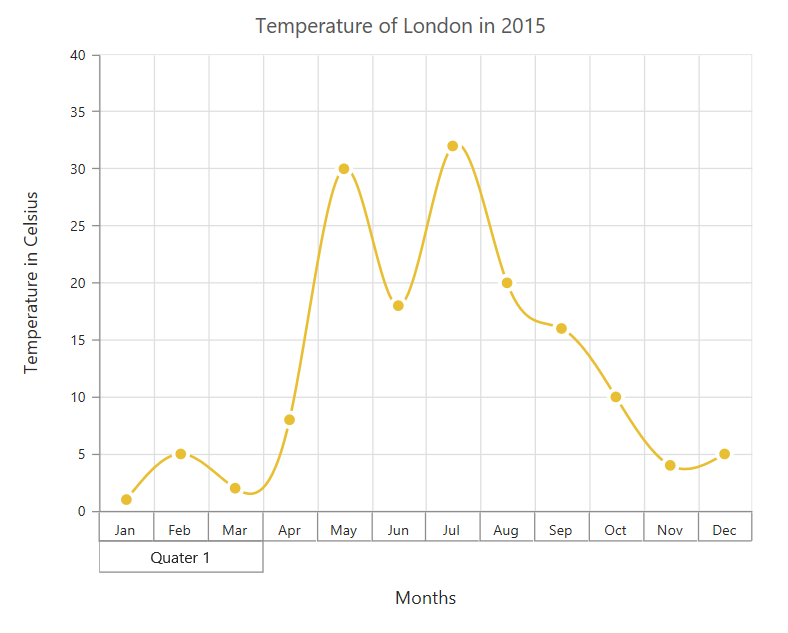### Customizing the multi-Level labels

The text of the labels can be customized by using the Text property.

• CSHTML
• ``````<ej-chart id="container">
// ...
<e-primary-x-axis>
<e-multi-level-labels>
<e-multi-level-label text="Year - 2015">
</e-multi-level-label>
</e-multi-level-labels>
</e-primary-x-axis>
// ...
</ej-chart>``````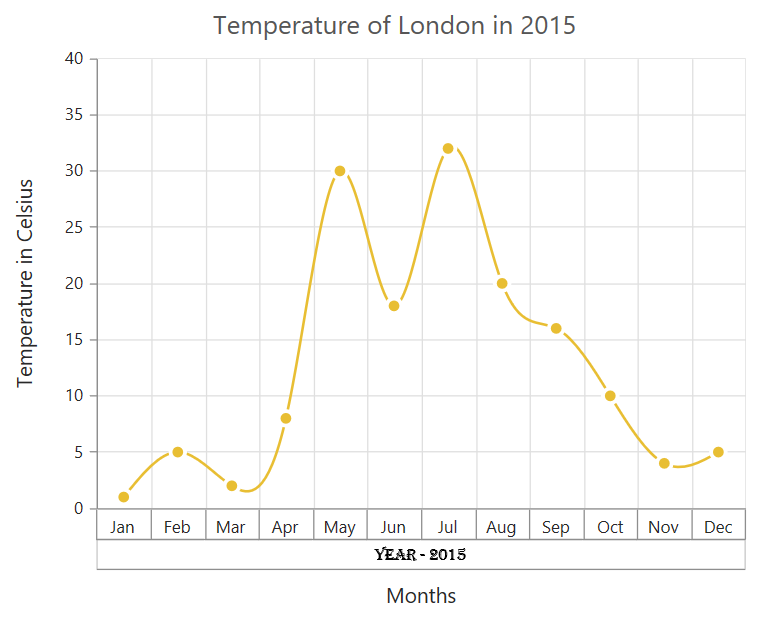You can change the alignment of the text to far, near, or center position by using the TextAlignment property. By default, the text will be aligned at center.

• CSHTML
• ``````<ej-chart id="container">
// ...
<e-primary-x-axis>
<e-multi-level-labels>
<e-multi-level-label text-alignment="Far">
</e-multi-level-label>
</e-multi-level-labels>
</e-primary-x-axis>
// ...
</ej-chart>``````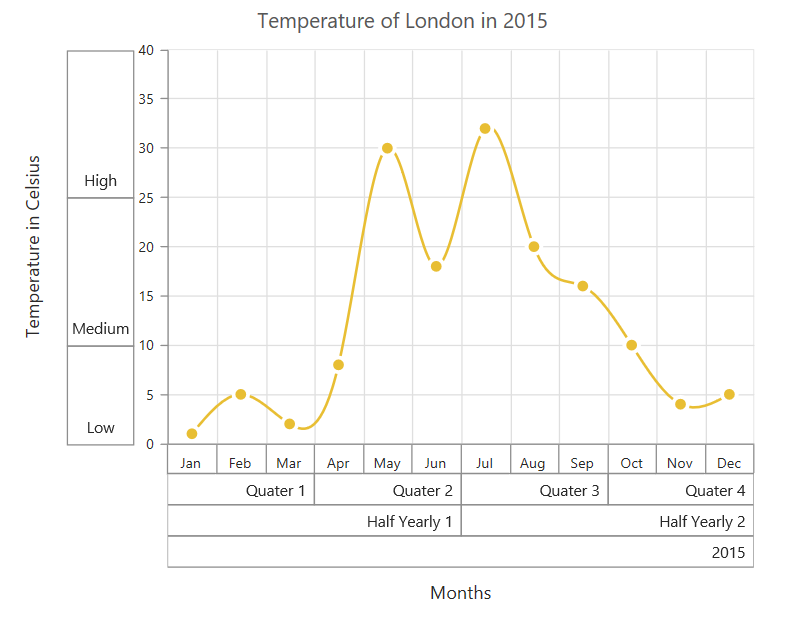You can trim, wrap, or wrapAndTrim the text if it exceeds the maximum text width value by using the TextOverflow property.

• CSHTML
• ``````<ej-chart id="container">
// ...
<e-primary-x-axis>
<e-multi-level-labels>
<e-multi-level-label text-overflow="Trim" maximum-text-width="40">
</e-multi-level-label>
</e-multi-level-labels>
</e-primary-x-axis>
// ...
</ej-chart>``````

The following screenshot illustrates the trimmed multi-level labels.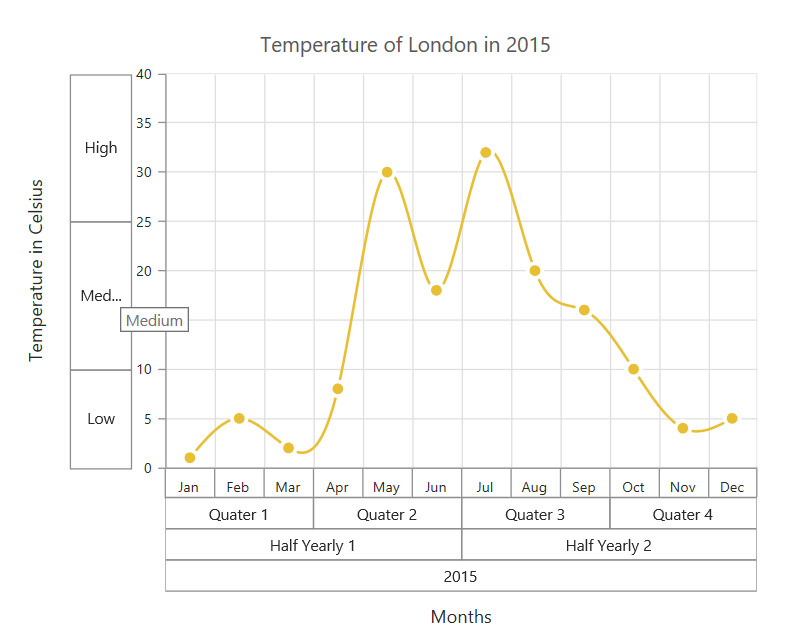And these labels can be placed in various rows by using the Level property.